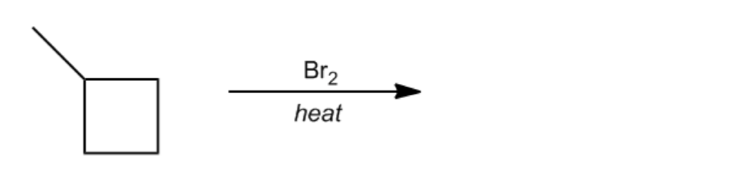Clutch Prep is now a part of Pearson
Ch. 11 - Radical ReactionsWorksheetSee all chapters

See all sections
Sections
Allylic Bromination

Most professors just want you to be able to determine the major product of a radical halogenation. However some are going to get a little more crazy!

Here I’ll teach you how to predict the exact percentages of monohalogenation products for differing halogens at room temperature. Again, only some professors will ask you for this. Be sure to refer to your class notes to see if this is something you should learn!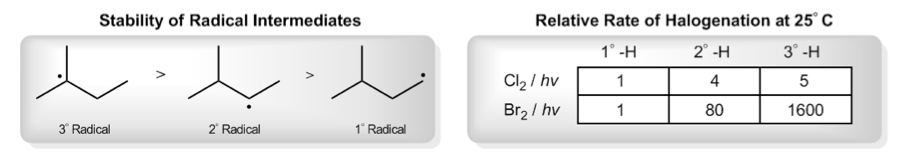Concept #1: Explaining relative rates of halogenation.

Transcript

All right guys, so in these videos, I'm going to be going through a much more vigorous explanation of how to calculate the major product for radical halogenation reaction. Now the reason that I'm even recording these videos is because your textbook goes through this more in-depth explanation and I want to make sure that we have all our bases covered.
Now understand that this might be beyond the scope of what many professors require for their students, so I'm going to leave it up to you to check with your professor to see if they want you to calculate these ratios or not. So let's just move right into the lesson.
Everyone knows by now the general trend of radical stability which is that tertiary radicals are more stable than secondaries, and secondaries are more stable than primaries. That's been enough for us so far. We've been able to get by and just predict the major product that way. But if we want to calculate the exact percentages of major and minor products, we're going to need equations. We're going to need some kind of quantitative method to do that.
And it turns out that we can calculate that knowing the relative rate of halogenation at a certain temperature. Here you see – this is a very important little table here. These are the relative rates of different types of halogenations at 25 degrees Celsius or room temperature. Let's just go through these numbers really quick, so you understand what it's meaning.
Remember how we discussed how chlorination was very unselective, kind of makes bad decisions everywhere and bromination makes these awesome decisions, well this can be quantified. The difference has to do with these halogenation rates. Notice that chlorination actually does prefer tertiaries. It has good intentions. The problem is that it doesn't prefer tertiaries very much, only slightly. It likes to halogenate tertiaries about five times more than primaries and only a little bit more than secondaries. You can see how chlorination, you're going to get a lot of products everywhere.
Now if you look at bromination, bromination is much more selective because bromination likes to brominate tertiary carbons 1,600 times more than primaries. You can imagine that that's why we call bromination highly selective versus chlorination which we basically call non-selective. It has to do with the fact that the difference between the relative rates are much bigger when you get to bromination.
Here we can go back to our definitions and we can state that chlorination is non-selective because the difference between the relative rates is very small, whereas bromination is highly selective because the difference between the relative rates is very large.
Now these ratios that I'm giving you here are only valid at one temperature and that's room temperature because that's the temperature that these experiments were conducted under. If we jack up the temperature for this reaction, the ratios between the different type of selectively become – can you guess? Smaller, meaning that there's less difference between primary, secondary and tertiary.
Why? The more heat you add to any reaction, the more ambient energy there's going to be, so the less selective it's going to be. It's going to wind up – even bromination can be coerced into some bad decisions at a high enough temperature.
Awesome. It's getting hot in here. That's basically the concept behind this table.
One note of caution for you guys, you might be flipping through your textbook and you might see slightly different values here. Like you might see it says 1 and then 4.2 and 5.5. I don't really care about the details. The reason I chose these numbers is because these are the safest, roundest numbers that I could find from a combination of sources. I looked online. I looked through a few different textbooks. And these numbers just seemed to make the most sense. There's no point in teaching you with complicated numbers. But, in general, the ranges are correct. It's 1, 4, 5, about there and then about 1, 80, 1,600.
If your professor teaches you different values, by all means, do not argue with him, just go with his values. Everything that I'm saying is still going to pertain to that, even if you have to use different values.

Example #1: Draw all of the monochlorination products of butane. Calculate percentage yields of each product.

Transcript

All right guys, let's go through this question. It says draw all monochlorination products of butane and calculate the percentage yields of each product. First of all, I just want to show you this table that I made. This table is going to make it very easy to calculate stuff just by filling in blank spaces. But the point isn't that you should have this at your exam, the point is that you should learn through this and then be able to do it on your own. We'll take this one as a worked example.
First of all, butane looks like that bolded molecule there. And the first thing we have to do is you have to calculate or count up the different types of protons that you have because remember that every type of proton has a different relative rate.
So as we can see I split it up into primary protons and secondary protons. What we can see is that after counting them up, you actually have six primary H's and you have four secondary H's. Does that make sense so far? Pretty easy. Now notice that there's no slot for tertiary, that is because there's no tertiaries here. But if I did have tertiaries, I'd have to add another row. Makes sense, right? Awesome.
So now we're going to plug in the relative rate. Where do I get that number? From my table. Yeah, relative rate of primary chlorination, see it says monochlorination. That's where this becomes important. I'm going to look at the numbers and it's 1 and 4, so I'm going to put that in here. 1 and 4. Wait. No, that's right. That's right. Perfect. Awesome. So I have 6 at a rate of 1. 4 at a rate of 4.
Now what I do is I multiply these numbers together. So what I'm going to get is – I'm going to get 6 here, so 6 times 1 equals 6. That's the relative reaction. Then 4 times 4 is 16. That means that literally, I'm getting that ratio – you see 6 over 16, that's going to be the final answer, but I need to put this in a percentage yield way.
The way we do that is we add up these two numbers. That means that the sum of these two numbers is 22. That's going to be the number that I put in my denominator. I'm going to put 22 as the bottom of my fraction for each one. Then for the top, I'm just going to put the actual relative amount. That means that 6 out of 22 went to the primary, so the fraction yield was 6 over 22. The fraction yield of my secondaries was 16 over 22.
Now these ratios came up a little bit too difficult to calculate in our heads, so let's use a calculator. You could also use – this is pretty simple math, so you could also use your iPhone if you wanted to. I'm an iPhone guy, sorry. So 6 divided by 22 gives me 27.2% or .3, let me round. Then 16 divided by 22 gives me 72.7%. Okay, that looks good.
So now I draw my products out. What you can see is that for my primary product where I get a chlorine here, I'm going to get 27.3% of that one. And then for my secondary where I put my chlorine here, I'm going to get 72.7% of that.
Now notice that chlorination in this case actually was fairly selective. 72% went for the better option, but still, when you're talking chemistry terms, this is not good enough and this difference is enough for a professor to say non-selective because no professor or no chemist wants to get 27% of their product as a waste. That would be considered a low-yield reaction. Even though it does seem kind of selective, it's actually not as selective as we need it to be to make this reaction worthwhile. Got it? Awesome.
Hopefully, this little layout that I made helps it to make sense. Eventually, I want you guys to be able to do this without a layout, just know what the next steps are. But for right now, let's go ahead and do some practice problems, where in the first one I'll help you along and then in the second one I'll take the training wheels off. Let's go ahead and work on the first problem.

By multiplying the number of equivalent hydrogens by its relative rate of halogenation, we can obtain a “relative reaction” rate. We compare the relative reactions to determine the percentage breakdown for each product.

I’ve made it really easy for you by building and filling out this chart. Eventually you should be able to fill in this chart yourself ;)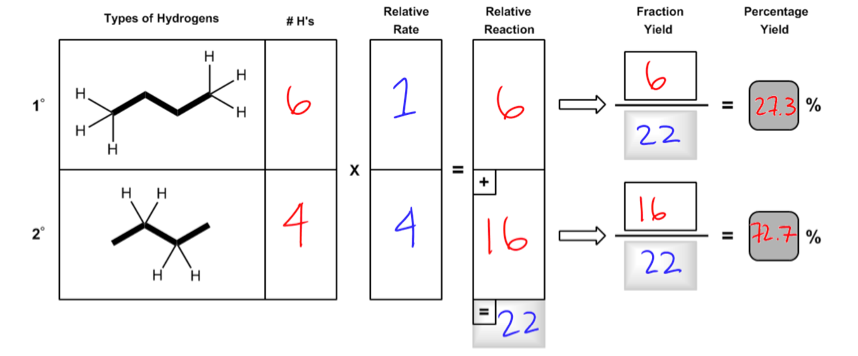The percentages we yield correspond to the amount of each product we would expect if we were to run this halogenation ourselves.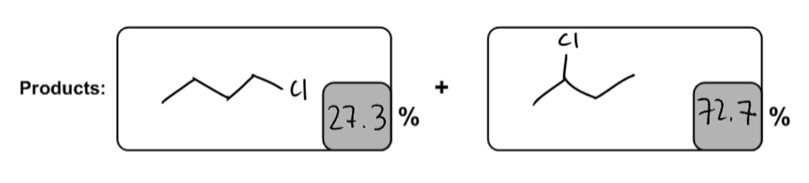Practice: What is the percentage yield of the major product?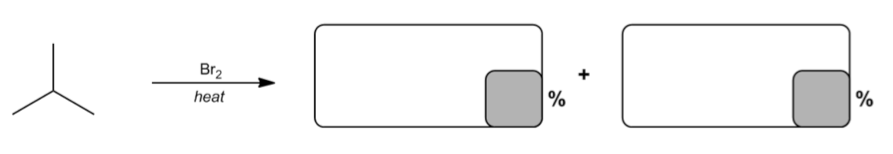Practice: What is the percentage yield of the major product?